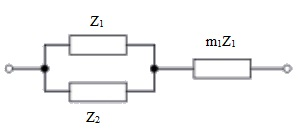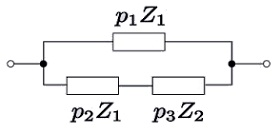#### Equivalent Impedance Transforms, Physics tutorial

Introduction to Equivalent Impedance Transforms:

We can state that equivalent impedance is an equivalent circuit of the electrical network of impedance elements that presents the similar impedance among all the pairs of terminals as did the given network and we now explain the arithmetic transformations between some passive, linear impedance networks generally found in the electronic circuits.

Some of the equivalent circuits in the linear network analysis comprise resistors in series, resistors in parallel and the extension to series and parallel circuits for capacitors, inductors and general impedances.

We emphasize the Thevenin's and Norton equivalent current generator and voltage generator circuits correspondingly and a good illustration is the Y-Δ transform.

That the number of equivalent circuits which a linear network can be converted into unlimited; even by the most trivial cases is true. For illustration, you might inquire how many various combinations of resistors in parallel are equivalent to a specific combined resistor and your answer is that it is virtually limitless.

A man named Wilhelm Cauer found out a transformation that could produce all the possible equivalents of a given rational, passive, linear one-port, or in another words, any given two-terminal  impedance. Transformations of 4-terminal, particularly 2-port, networks are as well generally found out and transformations of yet more complicated networks are possible.

The general nature of the subject of equivalent circuits is illustrated via Ronald Foster and George Campbell's in the year 1920 paper on non-dissipative four-ports. In the course of their work they looked at the manners four ports could be interconnected by means of ideal transformers and found a number of combinations that might have practical applications. If they tried to compute each and every single one of them, they arrived at a huge total of 83,539 equivalents.

2-Terminal, 2-Element Networks:

Single impedance consists of two terminals to join to the outside world; therefore you can explain it as a 2-terminal, or a one-port, network. In spite of this simple explanation, it might shock you that there is no limit to the number of meshes, the difficulty and number of elements that the impedance network might have.

2-element networks are very common in circuit design and we will find out that the filters, for instance, are frequently LC networks. Practically, circuit designers prefer RC networks as inductors of the LC networks are more complex to manufacture that RC networks.

Transformations of 2-element networks are simpler and are easier to determine than for 3-element networks. Take note that One-element networks can be assumed of as a special case of two-element network.

As it is possible to make use of the transformations on certain 3-element networks by replacing a network of elements for element Zn, you will be restricted to the replacement of a maximum of two impedances.

3-Element Networks:

As one-element networks, and two-element, two-terminal networks that include either two elements in series or two elements in parallel are trivial, the smallest number of elements which  a network that is non-trivial  might have is three and there are two 2-element-kind non-trivial transformations possible, one being both the reverse transformation and the topological dual, of the other.

Case I: Now, consider a 3-element network, its topological dual and its network's transform equations.Transform Equations:

p1 = 1 + m1

p2 = m1 (1 + m1)

p3 = (1 + m1)2

Transformed Network (Topological Dual):Case II: Now take a view at how you can begin from the topological dual network and derive its reverse transform:Transform Equations:

p1 = m12/(1 + m1)

p2 = m1/(1 + m1)

p3 = [m1/(1 + m1)]2Equivalent Circuit:

We can state with no contradiction that an equivalent circuit is the easiest form of a circuit which retains all of the electrical features of the original (and more complicated) circuit.

In its most general form, an equivalent circuit is build up of linear, passive elements. Though, more complex equivalent circuits are employed that approximate the nonlinear behavior of the original circuit as well.

Such more complicated circuits are frequently termed as macro models of the original circuit.  Operational amplifier is an extremely good illustration of the macro model.

There are two very significant two-terminal equivalent circuits you must know about:

Thevenin's equivalent: This decreases a two-terminal circuit to a single voltage source and series Thevenin's impedance

Norton equivalent: The further Element Theorem (EET) is the analytic method for simplifying the method of deriving driving point and transfer functions for linear electronic circuits  and as we will observe, it functions quite  similar to Thevenin's theorem  in that the additional element theorem breaks down one complex problem into some simpler ones that  decreases a two terminal circuit to a current source and a parallel Norton impedance.

For a limited set of linear four-terminal circuits, we can set up equivalent two-port networks. The limit on a two-port representation is that of a port: the current entering each and every port should be similar as the current leaving that port through linearizing a nonlinear circuit about its operating point; you can derive this two-port representation for transistors. We encompass two good illustrations of this representation in hybrid pi and h-parameter transistor equivalent circuits.

By mode of analogy we can as well make equivalent circuits that explain and model the electrical properties of materials or biological systems. The biological cell membrane for instance can be modeled as a lipid bi-layer capacitor in parallel having resistance-battery combinations that represent ion channels powered through an ion gradient across the membrane. Quite an interesting analogy would you state.

Extra Element Theorem:

The Extra Element Theorem (EET) is an analytic method for simplifying the method of deriving driving point and transfer functions for linear electronic circuits and as we will state, it functions quite similar to Thevenin's theorem in that the extra element theorem breaks down one complex problem into some simpler ones.

We can generally determine Driving point and transfer functions by using the processes of Kirchhoff's Voltage Law and Kirchhoff's Current Law. We might though discover that some of the complex equations can outcome that provide little insight into a circuit's behavior. By employing the extra element theorem, you can get rid of a circuit element like a resistor from a circuit and then you can determine the desired driving point or transfer function.

By eliminating the element that most complicates the circuit, similar elements that makes feedback, you can get the desired function more simply. Then the two correctional factors should be found and joined by the prior derived function to find out the precise expression.

The general form of the additional element theorem is termed as the N-extra element theorem and lets multiple circuit elements to be eliminated at once.

We will make use of driving point impedances to describe a special case of the Extra Element Theorem to determine the input impedance of a network.

Now, we write the Extra Element Theorem as represented below:

Zin = Zin [{1 + (Zeo/Z)}/{1 + (Ze/Z)}]

Here,

Z = Impedance selected as additional element.

Zin = Input impedance having Z removed (or made infinite)

Zeo = the impedance seen via the extra element Z having the input shorted (or made zero)

Ze= the impedance seen via the extra element Z having the input open (or made infinite)

If we calculate these three terms, it might seem similar to extra effort; however be rest assured that they are often simpler to calculate than the total input impedance.

Felici's Law:

We can for all time make use of Felici's law if we wish for to compute the total charge via a circuit configuration in which there is a current induced through a variable magnetic field. By Felici's law this is possible to compute the total charge in a period by employing initial and final flux for a conductor coil immersed in the variable magnetic field.

q(t) = (1/R) [Φ(0) - Φ(t)]

Let us explain this as shown below:

q(t) = ot i(τ) dτ = (1/R) ot fem (τ)dτ = (1/R)[Φ(0) - Φ(t)]

This will be simple for you to comprehend that if you recall that:

fem (τ) = - dΦ(t)/dt

Foster's Reactance Theorem

Foster's reactance theorem is significant for us in electrical network analysis and synthesis. This theorem defines that the reactance of a passive, lossless two-terminal (one-port) network always monotonically rises by means of frequency.

As strange as the statement above might come out to you, the proof of it was first presented in the year 1924 via Ronald Martin Foster; from which the law derives its name.

Reactance is the imaginary portion of the complex electrical impedance. The specification that the network should be passive and lossless means that there are no resistors (lossless), or amplifiers or energy sources (or passive) in the network. The network thus must comprise totally of inductors and capacitors and the impedance will be purely an imaginary number having zero real part. Other than that, the theorem is quite general; in specific, this applies to distribute element circuits however Foster formulated it in terms of discrete inductors and capacitors. Foster's theorem applies equivalently to the admittance of a network that is the susceptance (imaginary portion of admittance) of a passive, lossless one-port monotonically rises by the frequency.

Once again, this result might seem counterintuitive to you as admittance is the reciprocal of the impedance, however it is simply proved.

Now we prove it altogether.

When an impedance, Z = iX

Here,

Z is the impedance

X is the reactance

i is the imaginary unit

then the admittance is represented by:

Y = 1/iX = -i(1/X) = iB

Here,

B = susceptance

If X is monotonically rising having frequency then 1/X should be monotonically reducing. -1/X should as a result be monotonically rising and therefore it is proved that B is as well rising.

This is frequently the case in network theory that a principle or method apply uniformly to impedance or admittance as they do here. This is convenient in these conditions to make use of the concept of immittance that signify either impedance or admittance.

Foster's theorem can as well be defines in a more general form as, Foster's theorem (immittance form):

The imaginary immittance of a passive, lossless one-port monotonically rises by means of frequency.

Tutorsglobe: A way to secure high grade in your curriculum (Online Tutoring)

Expand your confidence, grow study skills and improve your grades.

Since 2009, Tutorsglobe has proactively helped millions of students to get better grades in school, college or university and score well in competitive tests with live, one-on-one online tutoring.

Using an advanced developed tutoring system providing little or no wait time, the students are connected on-demand with a tutor at www.tutorsglobe.com. Students work one-on-one, in real-time with a tutor, communicating and studying using a virtual whiteboard technology.  Scientific and mathematical notation, symbols, geometric figures, graphing and freehand drawing can be rendered quickly and easily in the advanced whiteboard.

Free to know our price and packages for online physics tutoring. Chat with us or submit request at info@tutorsglobe.com